• 概率论中的常用分布(用于考试前记忆) 离散情形 二项分布 描述：假设进行 nnn 次试验，每次试验成功的概率是 ppp ，失败的概率是 1−p1-p1−p .令随机变量 XXX 为成功的次数，则 XXX 服从二项分布.P(X=k)=(nk)pk(1...
概率论中的常用分布(用于考试前记忆)
离散情形

二项分布

描述：假设进行 $n$ 次试验，每次试验成功的概率是 $p$ ，失败的概率是 $1-p$ .令随机变量 $X$ 为成功的次数，则 $X$ 服从二项分布.$\mathbb{P}(X=k)=\binom{n}{k}p^k(1-p)^{n-k}$且有$\mathbb{E}(X)=np; Var(X)=np(1-p).$

几何分布

描述：假设进行 $n$ 次试验，每次试验成功的概率是 $p$ ，失败的概率是 $1-p$ .令 $X$ 表示直到第一次成功所需的试验次数，则 $X$ 服从几何分布.$\mathbb{P}(X=k)=p(1-p)^k$且有$\mathbb{E}(X)=\frac{1}{p}; Var(X)=\frac{1-p}{p^2}.$

负二项分布

描述：假设进行 $n$ 次试验，每次试验成功的概率是 $p$ ，失败的概率是 $1-p$ .令 $X$ 表示直到成功 $r$ 次所需的试验次数，则 $X$ 服从负二项分布.$\mathbb{P}(X=k)=\binom{k-1}{r-1}p^r(1-p)^{k-r}$且有$\mathbb{E}(X)=\frac{r}{p}; Var(X)=\frac{r(1-p)}{p^2}.$

泊松分布

描述：用于近似二项分布.$\mathbb{P}(X=k)=\frac{\lambda^ke^{-\lambda}}{k!}$且有$\mathbb{E}(X)=Var(X)=\lambda.$

超几何分布

描述：假设盒子中有 $n$ 个球，$r$ 个黑球，$n-r$个白球，从盒子中取出 $m$ 个球.令 $X$ 表示抽到的黑球数，则 $X$ 服从超几何分布.$\mathbb{P}(X=k)=\frac{\binom{r}{k}\binom{n-r}{m-k}}{\binom{n}{m}}$且有$\mathbb{E}(X)=m\frac{r}{n}(类似于二项分布的期望)$
连续情形

均匀分布

略

正态分布
pdf
$f(x)=\frac{1}{\sigma\sqrt{2\pi}}e^{-\frac{1}{2\sigma^2}(x-\mu)^2}$

指数分布
pdf
$f(x)=e^{-\lambda x}$且有$\mathbb{E}(X)=\frac{1}{\lambda},Var(X)=\frac{1}{\lambda^2}$

$\Gamma$分布、$\beta$分布、$\chi^2$分布一般不要求记忆，从略.


展开全文• 概率论中的关键定理的理解 楼主作为小白最近再搞强化学习方面的项目，发现和当初初学机器学习一样，概率论是一个永恒的话题，痛定思痛决定好好看下概率论及梳理统计相关的基础知识，写的比较零散，后续会跟进这些...
对概率论中的关键定理的理解
楼主作为小白最近再搞强化学习方面的项目，发现和当初初学机器学习一样，概率论是一个永恒的话题，痛定思痛决定好好看下概率论及梳理统计相关的基础知识，写的比较零散，后续会跟进这些理论在机器学习及预测控制上的应用（等我学会了，我认为强相关，立贴为证！）
贝叶斯公式的目的： 已知某事件已经发生，考察引发该事件的各种原因或情况的大小（P（Ai|B））
样本量足够大，概率比较小的二项分布，近似于泊松分布
寿命多服从指数分布
一个随机变量收到许多随机因素的影响，并且其中的每一个因素都不起主导作用，则符合正太（高斯）分布
指数分布的特点是，平均寿命就是该分部的参数
柯西分布特征
随机变量函数的期望等于变量期望的函数–很重要的行之，计算期望时直接复用随机变量的概率分布计算期望
大量小概率事件的分布符合泊松分布：查下该分布的起源
标准化定理：可以变量的数值归一化，从而削弱不同量级数值分布带来的影响
多维随机分布：多特征随机分布，应对特征间相关联的分布的定义和分析
大数定理：随机现象的平均结果的稳定性-大数定理
切比雪夫不等式：随机变量分布未知的情况下，时间围绕期望的下限估计
概率很小的随机事件，在个别试验中几乎不会发生-小概率原理
中心极限定理： 随机变量和的极限分布式正太分布
最大似然估计：如何根据样本，在假设的概率分布的基础上对参数进行“最大似然化”的估计
两个随机变量的协方差表征两个随机变量分布趋势的趋同度：首先协方差本身是要描述两个随机变量之间的关系，其次
譬如：相互独立时，两者共同发生的概率没有交合区，表征完全独立分布毫无关联，协方差对两个随机变量关系的反映，是通过进一步计算得到的相关系数来反应的：同时也表征了两个变量之间的线性相关程度！


展开全文动态规划
• 最近在看信息论中的概念，对于联合熵的概念比较混乱，从而引发出对联合概率的思考， 当提到联合概率的时候大家都会看到这么一...对于P(A,B)指的是在(A,B)形成的空间中的概率，Sa和Sb分别是A和B的空间，则形成总空间为S
最近在看信息论中的概念，对于联合熵的概念比较混乱，从而引发出对联合概率的思考，

当提到联合概率的时候大家都会看到这么一个图：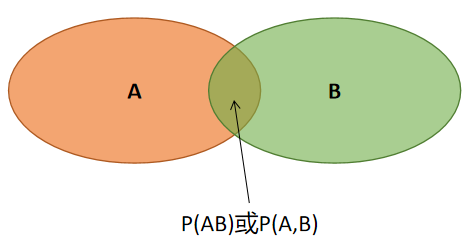学习概率论是这样理解还可以，还能解释条件概率，总体挺好的；

但是总感觉有点啥问题，当AB完全独立是不是这个：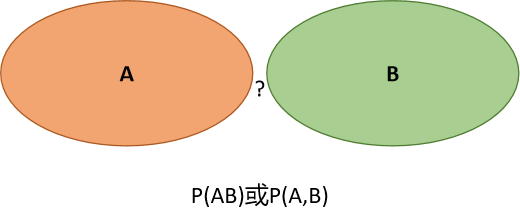这样就会随之想到的是P(AB)=0，书上又说A，B在相互独立时，P(AB)=P(A)*P(B)，是不是哪个地方有问题呢？

既然出现分歧，这就从概念出发吧。

对于P(A,B)指的是在(A,B)形成的空间中的概率，Sa和Sb分别是A和B的空间，则形成总空间为Sa*Sb。

假设A和B分别是线性空间，那么(A,B)就是二维平面。

P(AB)也可以写成P(AiBj)，或许这样就更好理解了。

举个例子，小明所穿的袜子，每星期内都随机穿，袜子有红黄绿蓝，星期几和穿袜子的颜色也是随机对应：

星期/颜色

红

黄

绿

蓝

1

-

-

-

-

2

-

-

-

-

3

-

-

-

-

4

-

-

-

-

5

-

-

-

-

6

-

-

-

-

7

-

-

-

-

P（红2）代表星期二穿红色的概率；

当两者相互独立时，P(红2)=P(红)*P(2)=1/4*1/7=1/28;

当小明在周六和周天不想穿绿色和蓝色，对于观察者进行大量实验后发现：

星期/颜色

红

黄

绿

蓝

1

-

-

-

-

2

-

-

-

-

3

-

-

-

-

4

-

-

-

-

5

-

-

-

-

6

- -

- -

7

- -

- -

此时发现P(红6)=P(红|6)*P(6)，其中P(6)的空间为整个空间，而P(红|6)的空间为6的空间；

此时观察者发现P(红|6)>P(红)说明红和6有某种关系，6使得红的概率增加；

同时发现P(绿|6)=0<P(红),说明6阻断绿的发生；

总之，用韦恩图代表概率容易让人混乱，联合时间并不是A和B同时发生的概率而是，A和B的特定组合占整个空间的概率；

所以P(AB)则衡量A和B空间联合的确定度，这样就和信息熵的概念一致了。

最后更正一下刚开始的图，相交的部分是P(A∩B)，而不是P(AB)：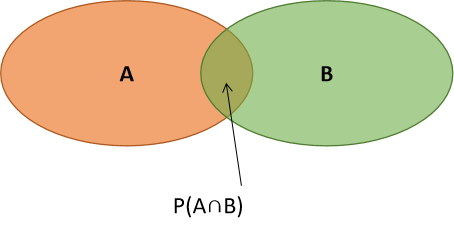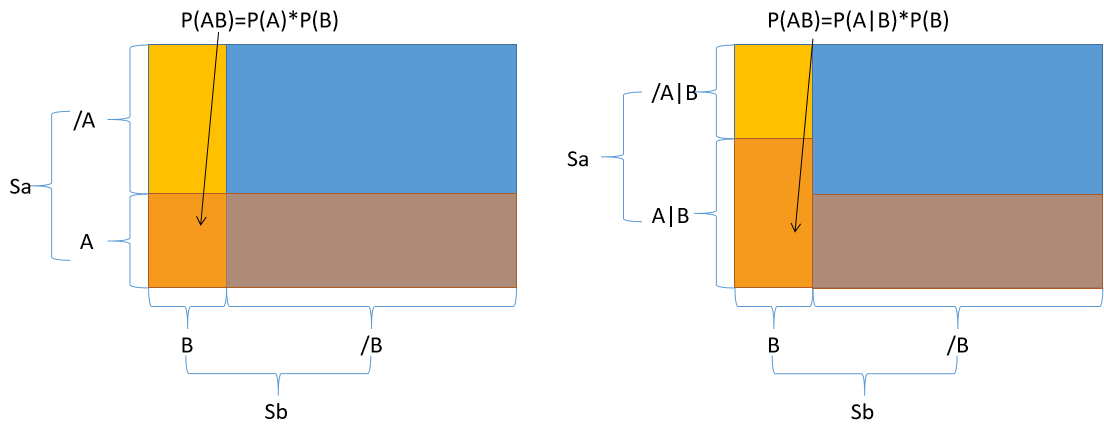展开全文机器学习 深度学习 人工智能
• P(B|A)=P(AB)P(A)P(B|A)=P(AB)P(A)P(B|A)=\frac {P(AB)}{P(A)} 2.乘法定理 P(AB)=P(B|A)P(A)P(AB)=P(B|A)P(A)P(AB)=P(B|A)P(A) 推广多个事件积事件 P(ABC)=P(C|AB)P(B|A)P(A)P(ABC)=P(C|AB)P(B...
1.条件概率

事件A已经发生的条件下，事件B发生
P(B|A)=P(AB)P(A)$P\left(B|A\right)=\frac{P\left(AB\right)}{P\left(A\right)}$P(B|A)=\frac {P(AB)}{P(A)}

2.乘法定理

P(AB)=P(B|A)P(A)$P\left(AB\right)=P\left(B|A\right)P\left(A\right)$P(AB)=P(B|A)P(A)
推广多个事件的积事件
P(ABC)=P(C|AB)P(B|A)P(A)$P\left(ABC\right)=P\left(C|AB\right)P\left(B|A\right)P\left(A\right)$P(ABC)=P(C|AB)P(B|A)P(A)
更一般地有
P(A1A2...An)=P(An|A1A2...An−1)P(An−1|A1A2...An−2)…P(A2|A1)P(A1)$P\left({A}_{1}{A}_{2}...{A}_{n}\right)=P\left({A}_{n}|{A}_{1}{A}_{2}...{A}_{n-1}\right)P\left({A}_{n-1}|{A}_{1}{A}_{2}...{A}_{n-2}\right)\dots P\left({A}_{2}|{A}_{1}\right)P\left({A}_{1}\right)$P(A_1A_2...A_n)=P(A_n|A_1 A_2...A_{n-1})P(A_{n-1}|A_1A_2...A_{n-2})\ldots P(A_2|A_1)P(A_1)

3.全概率公式

概念：
试验E的样本空间S，事件Bi${B}_{i}$$B_i$，i=1,2...,n$i=1,2...,n$$i = 1,2...,n$是样本空间的一个划分，每次试验有且仅有一个发生。

BiBj=∅,i≠j${B}_{i}{B}_{j}=\varnothing ,i\ne j$$B_iB_j=\varnothing, i \neq j$
B1∪B2∪...∪Bn=S${B}_{1}\cup {B}_{2}\cup ...\cup {B}_{n}=S$$B_1\cup B_2\cup...\cup B_n = S$

如果A是E的事件，事件A发生，
P(A)=P(A|B1)P(B1)+P(A|B2)P(B2)+...+P(A|Bn)P(Bn)$P\left(A\right)=P\left(A|{B}_{1}\right)P\left({B}_{1}\right)+P\left(A|{B}_{2}\right)P\left({B}_{2}\right)+...+P\left(A|{B}_{n}\right)P\left({B}_{n}\right)$P(A) = P(A|B_1)P(B_1) + P(A|B_2)P(B_2) + ... + P(A|B_n)P(B_n)

全概率公式的理解
例子：
人患肺癌的概率为0.1%，人群中有20%吸烟者，他们患肺癌的概率为0.4%, 那个不吸烟的人患肺癌的概率是多少？
换个人能看懂的说法，P(患肺癌)=0.001$P\left(患肺癌\right)=0.001$$P(患肺癌) = 0.001$,  P(吸烟)=0.2$P\left(吸烟\right)=0.2$$P(吸烟) = 0.2$,  P(患肺癌|吸烟)=0.004$P\left(患肺癌|吸烟\right)=0.004$$P(患肺癌|吸烟) = 0.004$, 求 P(患肺癌|不吸烟)=?$P\left(患肺癌|不吸烟\right)=?$$P(患肺癌|不吸烟) = ?$
P(患肺癌)=0.001$P\left(患肺癌\right)=0.001$$P(患肺癌) = 0.001$ 既包括了吸烟患肺癌的概率又包括不吸烟患肺癌的概率。
P(患肺癌)=P(吸烟患肺癌)+P(不吸烟患肺癌)$P\left(患肺癌\right)=P\left(吸烟患肺癌\right)+P\left(不吸烟患肺癌\right)$P(患肺癌) = P(吸烟患肺癌) + P(不吸烟患肺癌) =P(患肺癌|吸烟)P(吸烟)+P(患肺癌|不吸烟)P(不吸烟)$=P\left(患肺癌|吸烟\right)P\left(吸烟\right)+P\left(患肺癌|不吸烟\right)P\left(不吸烟\right)$= P(患肺癌|吸烟) P(吸烟) + P(患肺癌|不吸烟) P(不吸烟) =0.004×0.2+P(患肺癌|不吸烟)×(1−0.2)=0.001$=0.004×0.2+P\left(患肺癌|不吸烟\right)×\left(1-0.2\right)=0.001$= 0.004\times 0.2 + P(患肺癌|不吸烟) \times (1-0.2) = 0.001
所以不吸烟的人患肺癌的概率为0.00025.

4.贝叶斯公式

P(Bi|A)=P(A|Bi)P(Bi)∑nj=1P(A|Bj)P(Bj)$P\left({B}_{i}|A\right)=\frac{P\left(A|{B}_{i}\right)P\left({B}_{i}\right)}{\sum _{j=1}^{n}P\left(A|{B}_{j}\right)P\left({B}_{j}\right)}$P(B_i|A) = \frac{P(A|B_i)P(B_i)}{\sum_{j=1}^{n} P(A|B_j)P(B_j)}
n=2时，
P(B|A)=P(A|B)P(B)P(A|B)P(B)+P(A|B¯¯¯¯)P(B¯¯¯¯)$P\left(B|A\right)=\frac{P\left(A|B\right)P\left(B\right)}{P\left(A|B\right)P\left(B\right)+P\left(A|\overline{B}\right)P\left(\overline{B}\right)}$P(B|A) = \frac{P(A|B)P(B)}{P(A|B)P(B)+P(A| \overline B)P(\overline B)}

用条件概率、全概率公式理解贝叶斯公式：
P(A|B)=P(A∩B)P(A)$P\left(A|B\right)=\frac{P\left(A\cap B\right)}{P\left(A\right)}$P(A|B) = \frac{P(A\cap B)}{P(A)}或者
P(B|A)=P(B∩A)P(A)$P\left(B|A\right)=\frac{P\left(B\cap A\right)}{P\left(A\right)}$P(B|A) = \frac{P(B\cap A)}{P(A)}因为P(A∩B)=P(B∩A)$P\left(A\cap B\right)=P\left(B\cap A\right)$P(A\cap B)= P(B\cap A)所以P(B|A)=P(A|B)P(B)P(A)$P\left(B|A\right)=\frac{P\left(A|B\right)P\left(B\right)}{P\left(A\right)}$P(B|A) = \frac{P(A|B)P(B)}{P(A)}
P(A)发生的概率就用到了全概率公式，包括B在各种情况下A发生的概率：∑j=1nP(A|Bj)P(Bj)$\sum _{j=1}^{n}P\left(A|{B}_{j}\right)P\left({B}_{j}\right)$\sum_{j=1}^{n} P(A|B_j)P(B_j)

实际应用中的定义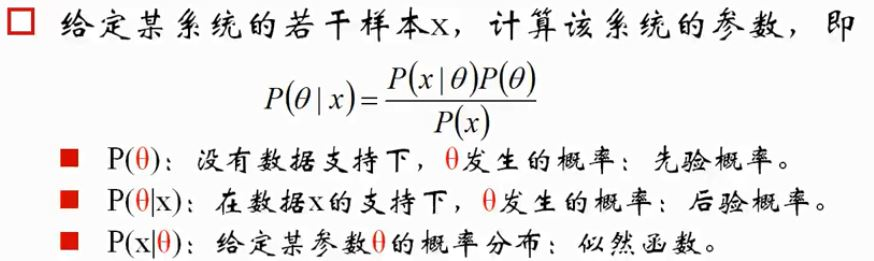经典例子：
癌症诊断事件，人患癌症的统计概率为0.005，一个不患癌症的受诊者试验呈阳性的概率为0.05，一个患癌症的病人做诊断时呈阳性的概率为0.95，那么受诊者试验呈阳性，他患癌症的概率？
分析：
P(患癌症)=0.005$P\left(患癌症\right)=0.005$$P(患癌症) = 0.005$
P(呈阳性|不患癌症)=0.05$P\left(呈阳性|不患癌症\right)=0.05$$P(呈阳性|不患癌症) = 0.05$
P(呈阳性|患癌症)=0.95$P\left(呈阳性|患癌症\right)=0.95$$P(呈阳性|患癌症) = 0.95$
P(患癌症|呈阳性)=?$P\left(患癌症|呈阳性\right)=?$$P(患癌症|呈阳性) = ?$

使用条件概率计算：
P(患癌症|呈阳性)=P(呈阳性|患癌症)P(患癌症)P(呈阳性)$P\left(患癌症|呈阳性\right)=\frac{P\left(呈阳性|患癌症\right)P\left(患癌症\right)}{P\left(呈阳性\right)}$P(患癌症|呈阳性) =\frac{P(呈阳性|患癌症)P(患癌症)}{P(呈阳性)}P(呈阳性|患癌症)P(患癌症)=0.005×0.95=0.00475$P\left(呈阳性|患癌症\right)P\left(患癌症\right)=0.005×0.95=0.00475$ P(呈阳性|患癌症)P(患癌症) = 0.005 \times 0.95 = 0.00475P(呈阳性)=P(呈阳性|不患癌症)P(不患癌症)+P(呈阳性|患癌症)P(患癌症)$P\left(呈阳性\right)=P\left(呈阳性|不患癌症\right)P\left(不患癌症\right)+P\left(呈阳性|患癌症\right)P\left(患癌症\right)$P(呈阳性) = P(呈阳性|不患癌症)P(不患癌症) + P(呈阳性|患癌症)P(患癌症) =0.05×(1−0.005)+0.95×0.005=0.0545$=0.05×\left(1-0.005\right)+0.95×0.005=0.0545$= 0.05\times(1-0.005)+0.95\times0.005= 0.0545P(患癌症|呈阳性)=0.004750.0545=0.08715$P\left(患癌症|呈阳性\right)=\frac{0.00475}{0.0545}=0.08715$P(患癌症|呈阳性) =\frac{0.00475}{0.0545} = 0.08715
展开全文条件概率 全概率公式 贝叶斯公式
• 其中：P(a, b)表示 a和b事件同时发生概率，P(a | b)是一个条件概率，表示在b事件发生条件下，a发生概率 3个事件概率链式调用： P(a, b, c) = P(a | b, c) * P(b, c) 　=P(a | b, c) * P(b | c) * P(c) ...
• 即等可能概型，空间包含有限个元素，每个元素出现概率是一样，例如掷硬币。 条件概率 P(B|A)表示在发生A情况下发生B。 独立性 P(AB)表示既发生了A也发生了B。P(AB)=P(A)P(B),则AB相互独立。 ***通常说分布...机器学习
• P（我当时学时候是A）表示排列方法,表示一些物体按顺序排列起来,总共方法是多少. 计算是固定套路,熟能生巧,多计算几个就熟练了. 举个例子,C（5,2）=（5*4）/（2*1）=10,C（7,3）=7*6*5 / 3*2*1=35 P（5,3
• ## 概率论中的独立性

千次阅读 2020-04-21 17:14:27
若随机事件A和事件B概率满足关系：P{AB}=P{A}*P{B} ，则称事件A和事件B是随机独立（或简称独立） 随机变量独立性 随机变量：定义在样本空间上函数（离散或连续） 在同一样本空间内，若随机事件A发生时...
• 有这样一道概率论题目，我认为将其编写为C程序实现是一件非常有意思事情，题目如下： 在一个人数很多团体中普查某种疾病，为此要抽验N...在概率论中，这是一道依照期望来比较题目，但当我们将它视为一道C语言题算法 c语言
• E, F分别表示某类事件，于是E可以表示成E=EF∪E(F^c)， 即E中的事件要么同时属于E和F，要么仅属于E而不属于F。 那么E事件发生的概率可表示为： 可以这样理解上述公式： 事件E发生的概率，等于 F发生时，E发生的...条件概率 贝叶斯公式
• 转载于:https://www.cnblogs.com/tuty/p/4882509.html
• A、B 发生概率分别为 P(A) 和 P(B) ， AB 事件同时发生概率为 P(AB) 若 P(A)×P(B)=P(AB) ，则 A 与 B 相互独立。事件 A 发生概率不影响事件 B 发生概率，反应是概率运算上关系。 不相关：不相关随机...
• 注：其实从中学就开始学习统计学了，最早的写"正"字唱票（相当于寻找众数），就是一种统计分析的过程。还有画直方图，求平均值，找位数等。自己在学校里并没有完整系统...从最初的p值的含义，到各种分布，假设检验...
• 连续型随机变量（or 连续型概率分布） ... P 概率（函数） P 概率（函数）、分布列、分布律 f 概率密度（函数）PDF PDF、PMF、CDF 区别详见https://blog.csdn.net/wzgbm/article/details/51680540...数学
• 机器学习中的概率论知识 古典概型 P(Aˉ)=1−P(A)P(\bar{A}) = 1-P(A)P(Aˉ)=1−P(A) P(A−B)=P(A)−P(AB)P(A-B) = P(A) - P(AB)P(A−B)=P(A)−P(AB) P(A+B)=P(A)+P(B)−P(AB)P(A+B) = P(A)+P(B)-P(AB)P(A+B)=P(A)+...
• 动态规划应用十分常见，今天介绍一种在概率论中求期望时所用动态规划方法。 因为在求期望时，我们需要知道N种结果中，每种结果概率p[i]和每种结果值k[i],那么最终期望值即为E=pk+pk+...p[N]k[N...
• 定义： ...如果P(B∣A)=P(B)P(B|A)=P(B)P(B∣A)=P(B)，则表明事件A对B无影响，即A和B是相互独立。 例：抛硬币2次，设A为第一次出现正面，B为第二次出现正面事件，则： P(A)=12P(A)=\frac{1}{2}P
• 在随机试验，可能出现也可能不出现，而在大量重复试验具有某种规律性事件 2.条件概率 假设实验A和B是试验E事件， 考虑A已发生情况下B发生概率： P(B∣A)=P(AB)/P(A)P(B|A)=P(AB)/P(A)P(B∣A)=P(AB)/P(A)...
• 一 事件与概率 概率空间 样本点：在试验每一...概率频率解释：设在相同条件下重复地进行试验，则随着试验次数地不断增大，事件A频率在某一确定值附近趋于稳定，这一确定值称为事件A概率，记为P(A)P(A)P(A)机器学习 统计学
• P(B|A)=13 表示意思为当A发生时候，B发生概率 公式： P(B|A)=P(AB)P(A) P(AB)=P(B|A)∗P(A)=P(A|B)∗P(B) P(A|B)=P(B|A)∗P(A)P(B) 全概率公式 B1,B2,B3……Bn 为样本空间S一个划分则可以得到 P(A)=P(B1)P...
• 若x为离散/连续变量，则P=(x=x0)表示x0发生概率/概率密度 1.2 累计分布函数 Φ(x)=P(≤x0) Φ(x)一定为单增函数 min(Φ(x))=0，max(Φ(x))=1 将值域为[0，1]某函数y=f(x)看成x事件累积概率 若y=f(x)可导，则...机器学习
• 独立：对于事件$A$和$B$，如果$P(AB)$=$P(A)P(B)$，那么称$A$和$B$是独立。 所谓独立，即两事件结果不会相互影响。从样本点⻆度来考虑，即两者不包含相同样本点。 条件概率 条件概率： 如果\$P(B)&gt...
• 泊松分布，要明白：泊松分布是二项分布n很大而p很小时一种极限形式。 二项分布：已知某件事情发生概率是p，那么做n次试验，事情发生次数就服从二项分布。 泊松分布式某段连续时间内事情发生次数。事情...
• 概率论中常见分布数学期望、方差与特征函数推导 （一）离散型分布 #1.单点分布 #2.两点分布 #3.二项分布 #4.泊松分布 #5.超几何分布 #6.几何分布 #7.负二项分布 1.单点分布 随机变量取值，X=a(常数) 分布律：P(X...统计学...